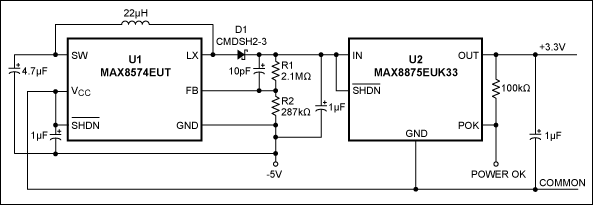# Miniature Circuit Converts Negative Inputs to Positive Outputs

### Abstract

You can obtain a precise, positive output voltage from a negative voltage supply by teaming a boost converter with a linear regulator. The input and output capabilities of the circuit depend on the allowable input/output voltages of ICs U1 and U2.

A similar version of this article appeared in the April 23, 2009 issue of EDN magazine.

You can obtain a precise, positive output voltage from a negative voltage supply by teaming a boost converter with a linear regulator as shown in Figure 1. The input and output capabilities of the circuit depend on the allowable input/output voltages of ICs U1 (MAX8574) and U2 (MAX8875). In this case, U1 and U2 are chosen to convert a -5V input voltage to a +3.3V output voltage.U1 is a boost converter that accepts -5V when its VCC pin is connected to the common ground (the ground of the negative power supply input). Voltage divider R1/R2 at the output of this U1 circuit provides the feedback necessary to set the output voltage 10.5V above U1's GND pin. With the FB threshold voltage factory-set to 1.226V, you can choose R1 and R2 using this equation: (1.226V/R2)(R1 + R2) = 10.5V. Current through the R1 and R2 resistors should be at least 2µA. The U1 output (U2's IN) is 10.5V above -5V, which is +5.5V with respect to the common ground.

U2, a linear regulator whose GND pin connects to the common ground, accepts input voltages up to +6.5V. Its output is factory set to +3.3V. Figure 2 shows the Figure 1 output voltage versus output current for input voltages of -4.5V, -5V, and -5.5V.